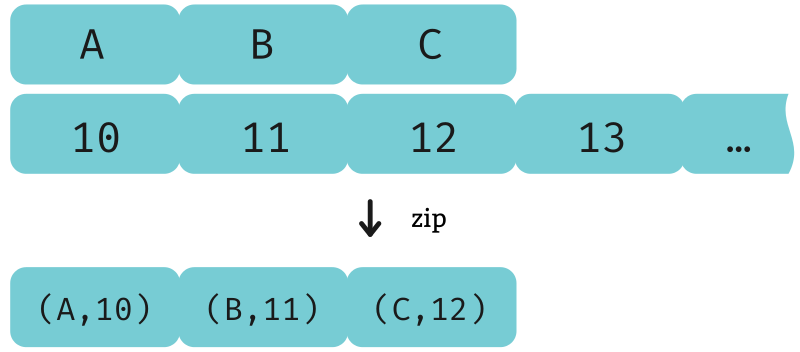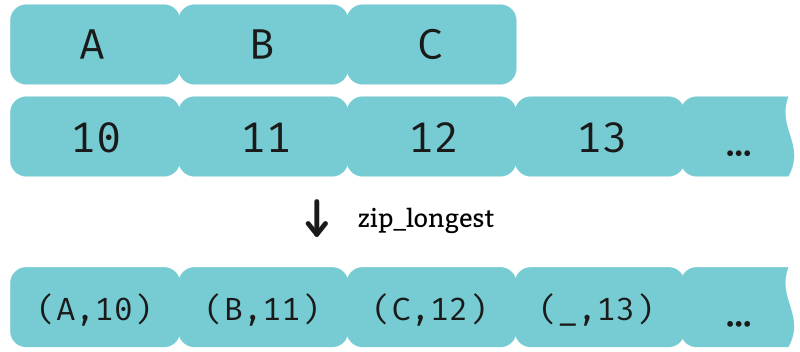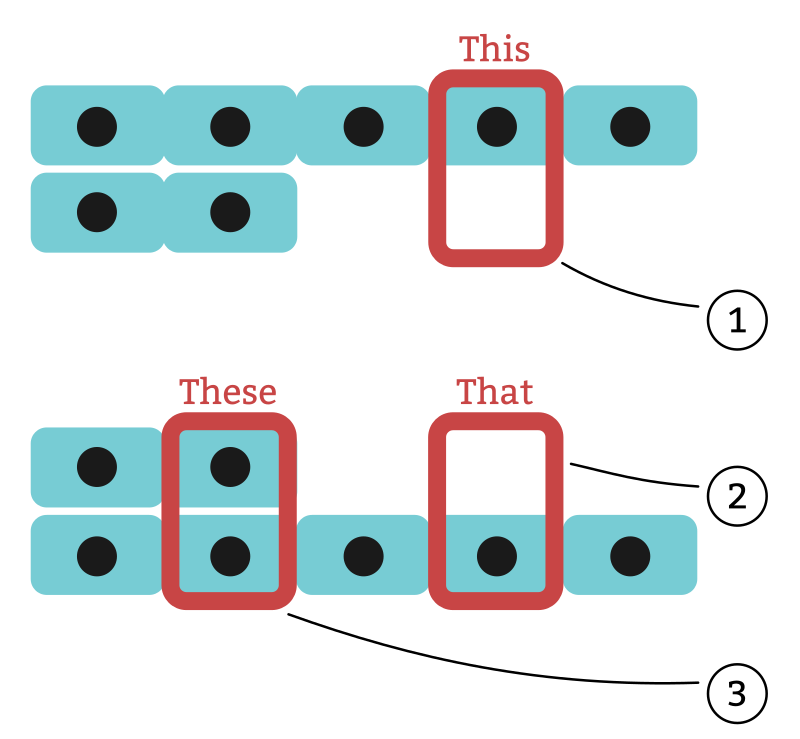# Zipping

Here we’ll look at these closely-related Python functions:

• `zip`
• `itertools.zip_longest`
• `enumerate`

• `zip`
• `zip3`, `zip4`, etc.
• `padZip`

## `zip`

Using Python’s built-in `zip` function `zip` in Python is a lot like using `map` with more than one iterable. The difference is that here we don’t give a function that specifies how to combine the list elements; instead, the list elements always get packed together in a tuple.

``````>>> it = zip('ABC', count(10))

>>> list(islice(it, 5))
[('A', 10), ('B', 11), ('C', 12)]``````Notice that when the input lists have different lengths, the resulting list’s length is the shorter of the two; the remaining elements from the longer input are discarded.

The corresponding Haskell function has the same name and the same result. `zip` in `Data.List` and `Prelude`

``````λ> xs = zip "ABC" (enumFrom 10)

λ> take 5 xs
[('A',10),('B',11),('C',12)]``````

### Zipping more than two lists

Python’s `zip` is variadic; you can give it as many lists as you want, and it will zip them all.

• Applying `zip` to three arguments gives you a list of 3-tuples,
• Applying `zip` to four arguments gives you a list of 4-tuples,
• And so on.
``````>>> it = zip([1,2], 'ab', ["one", "two"])

>>> list(it)
[(1, 'a', 'one'), (2, 'b', 'two')]``````

Haskell doesn’t generalize over function arity in this way, so instead we have a different function for each number of lists: `zip3`, `zip4`, etc. `zip3` in `Data.List` and `Prelude`

``````λ> zip3 [1,2] "ab" ["one", "two"]
[(1,'a',"one"),(2,'b',"two")]``````

Compare the types of these zip functions:

``````zip  :: [a] -> [b]               -> [(a, b)]
zip3 :: [a] -> [b] -> [c]        -> [(a, b, c)]
zip4 :: [a] -> [b] -> [c] -> [d] -> [(a, b, c, d)]``````

## `zip_longest`

We mentioned that `zip` handles inputs of different lengths by ignoring some elements of the longer inputs. `zip_longest` takes a different strategy: it pads the shorter list with `None` to fill in the gaps.

``>>> it = zip_longest('ABC', count(10))``

In this case, since `count(10)` is infinite, the result `it` is also infinite. In the REPL example below we truncate it with `islice` to show how it begins.

``````>>> list(islice(it, 5))
[('A', 10),
('B', 11),
('C', 12),
(None, 13),
(None, 14)]``````The Haskell `base` package doesn’t have a function akin to this. Instead we’ll look to a package called `semialign`, and specifically a module within it named `Data.Align`. This module expands greatly on the concept of zipping, generalizing it to a notion they call alignment which includes “zipping” things other than lists. We’ll stick to focusing on lists here, though. There’s a function called `padZip` `padZip` in `Data.Align` in the `semialign` package which is pretty similar to `zip_longest`:

``````λ> xs = padZip "ABC" (enumFrom 10)

λ> take 5 xs
[(Just 'A',Just 10),
(Just 'B',Just 11),
(Just 'C',Just 12),
(Nothing,Just 13),
(Nothing,Just 14)]``````

Notice the insertion of the `Just` constructors, because the values are now all lifted into `Maybe` to accommodate the possibility that any of them may be `Nothing`. Here are the type signatures for `zip` and `padZip` for comparison:

``````zip    :: [a] -> [b] -> [(a, b)]
padZip :: [a] -> [b] -> [(Maybe a, Maybe b)]``````

If all of these `None`s, `Nothing`s, and `Just`s aren’t to your liking, you may be interested in the `fillvalue` parameter.

### The `fillvalue` parameter

This named parameter on `zip_longest` lets you specify your own value with which to pad the shorter lists instead of `None`.

``````>>> it = zip_longest('ABCD', 'xy', fillvalue='-')

>>> list(it)
[('A', 'x'), ('B', 'y'), ('C', '-'), ('D', '-')]``````

The `semialign` package doesn’t have this exact function, `alignWith` in `Data.Align` in the `semialign` package but it has a few things we can put together to get this result. `fromThese` in `Data.These` in the `these` package

``````λ> alignWith (fromThese '-' '-') "ABCD" "xy"
[('A','x'),('B','y'),('C','-'),('D','-')]``````

We’ll take a moment to explain how we came up with this expression and what it means.

When you align a list of `a` and a list of `b`, for each list position there are three possibilities:1. We’ve gone past the end of the `b` list, so there is only an `a`;
2. We’ve gone past the end of the `a` list, so there is only an `b`; or
3. We haven’t reached the end of either list yet, and so there is both an `a` and a `b`.

These three possibilities are represented by the type called “These”, We discuss `These` more thoroughly in a Functortown lesson about bifunctors. which is where the `these` package gets its name.

``````data These a b = This a | That b | These a b
--                   (1)      (2)        (3)``````

Compare the type signature of `alignWith` to that of `zipWith` that we saw earlier:

``````zipWith   :: (a -> b -> c)    -> [a] -> [b] -> [c]
alignWith :: (These a b -> c) -> [a] -> [b] -> [c]``````

The only thing that has changed is the parameters to the function argument; a function that we use for zipping always receives both an `a` and a `b`, whereas a function that we use for aligning receives value of type `These a b` which can be either of the three possibilities we just discussed.

In this case, our goal (for the sake of emulating the Python result) was to produce a list of tuples, so `alignWith` specializes further to:

``alignWith :: (These a b -> (a, b)) -> [a] -> [b] -> [(a, b)]``

So we needed a function of type `These a b -> (a, b)`, and that’s exactly what `fromThese` does. We give it a default value to fill in the blank if the `a` is missing, another value to fill in if the `b` is missing (in this example, we used `'-'` for both), and `fromThese` turns a `These` into a tuple.

``fromThese :: a -> b -> These a b -> (a, b)``

## `enumerate`

The Python built-in `enumerate` function `enumerate` in Python zips a list with a sequence of incrementing numbers.

### Enumerating from zero

By default, the sequence starts with zero.

``````>>> it = enumerate(['zero', 'one', 'two'])

>>> list(it)
[(0, 'zero'), (1, 'one'), (2, 'two')]``````

Typically in Haskell we would express this using the `zip` function that we introduced earlier.

``````λ> zip (enumFrom 0) ["zero", "one", "two"]
[(0,"zero"),(1,"one"),(2,"two")]``````

### The `start` parameter

The `enumerate` function also has an optional second parameter called `start`, allowing the sequence to begin with some number other than zero.

``````>>> it = enumerate(['five', 'six', 'seven'], start = 5)

>>> list(it)
[(5, 'five'), (6, 'six'), (7, 'seven')]``````

And in the Haskell version, we would make this change by replacing `0` with the desired starting value.

``````λ> zip (enumFrom 5) ["five", "six", "seven"]
[(5,"five"),(6,"six"),(7,"seven")]``````Join Type Classes for courses and projects to get you started and make you an expert in FP with Haskell.